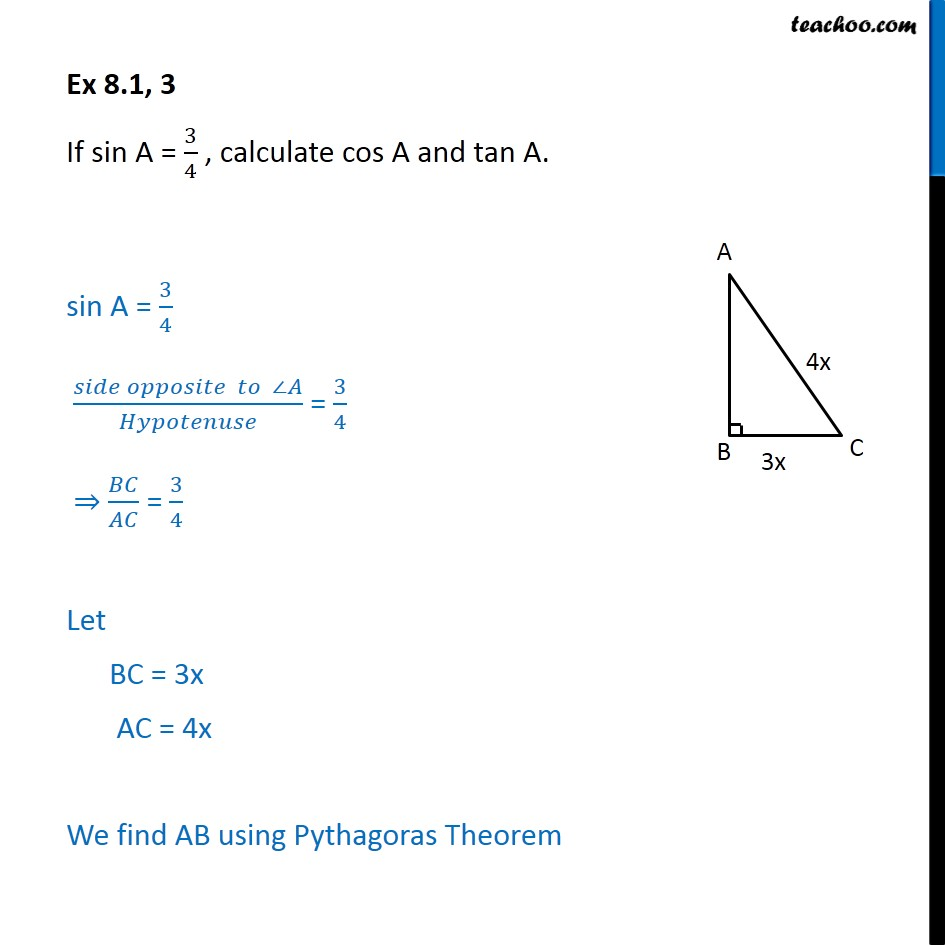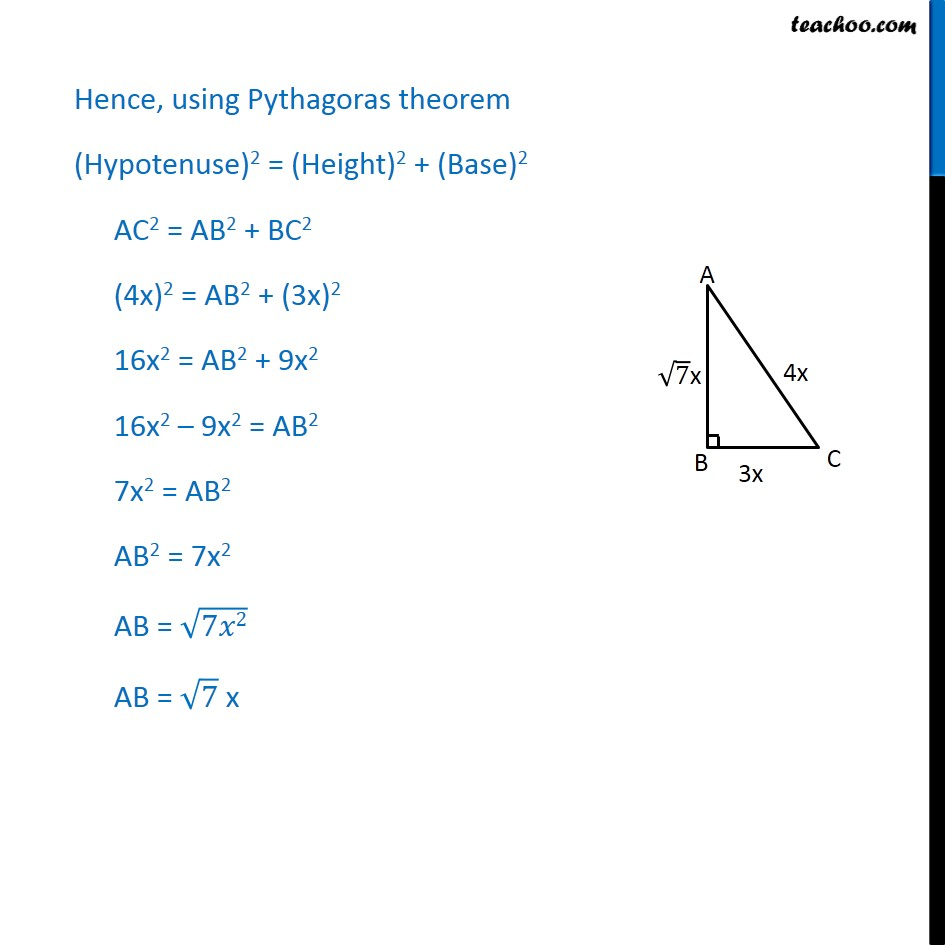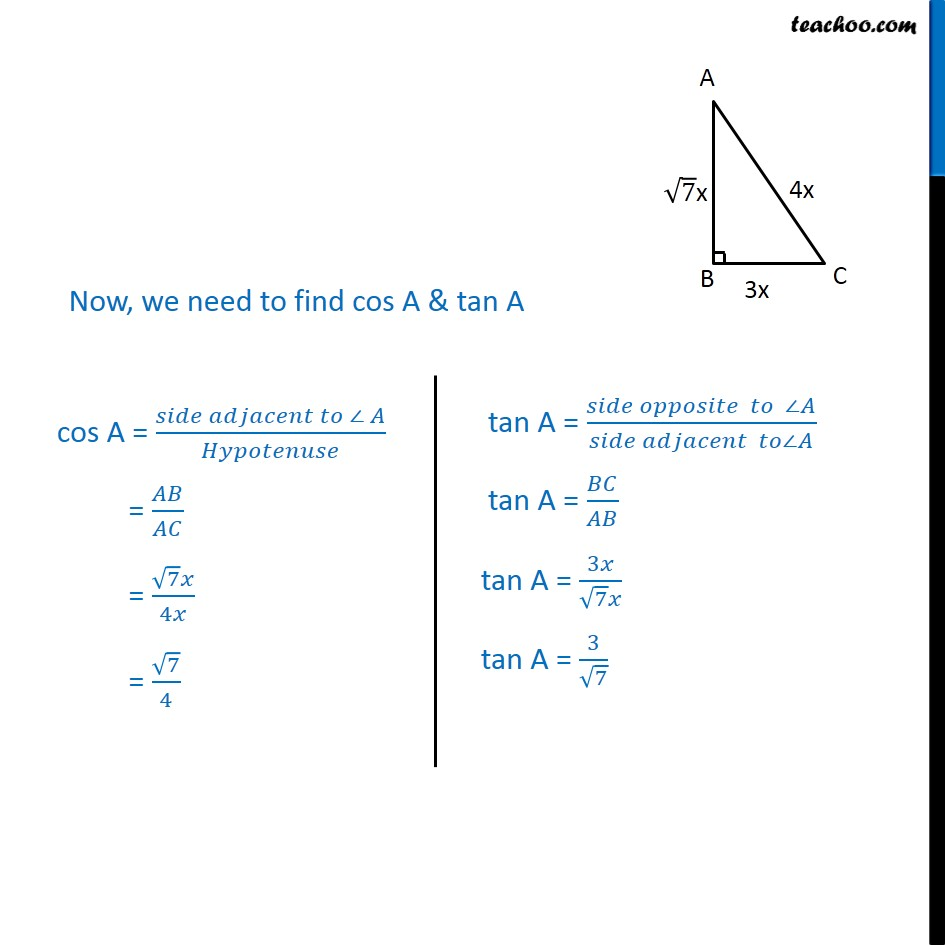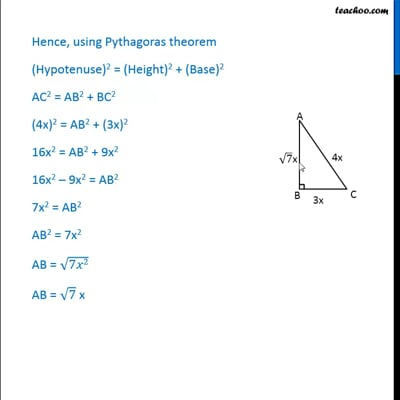Ex 8.1

Chapter 8 Class 10 Introduction to Trignometry
Serial order wiseThis video is only available for Teachoo black users

Solve all your doubts with Teachoo Black (new monthly pack available now!)

### Transcript

Ex 8.1, 3 If sin A = 3/4 , calculate cos A and tan A. sin A = 3/4 (𝑠𝑖𝑑𝑒 𝑜𝑝𝑝𝑜𝑠𝑖𝑡𝑒 𝑡𝑜 ∠𝐴)/𝐻𝑦𝑝𝑜𝑡𝑒𝑛𝑢𝑠𝑒 = 3/4 ⇒ 𝐵𝐶/𝐴𝐶 = 3/4 Let BC = 3x AC = 4x We find AB using Pythagoras Theorem Hence, using Pythagoras theorem (Hypotenuse)2 = (Height)2 + (Base)2 AC2 = AB2 + BC2 (4x)2 = AB2 + (3x)2 16x2 = AB2 + 9x2 16x2 – 9x2 = AB2 7x2 = AB2 AB2 = 7x2 AB = √7𝑥2 AB = √7 x Now, we need to find cos A & tan A